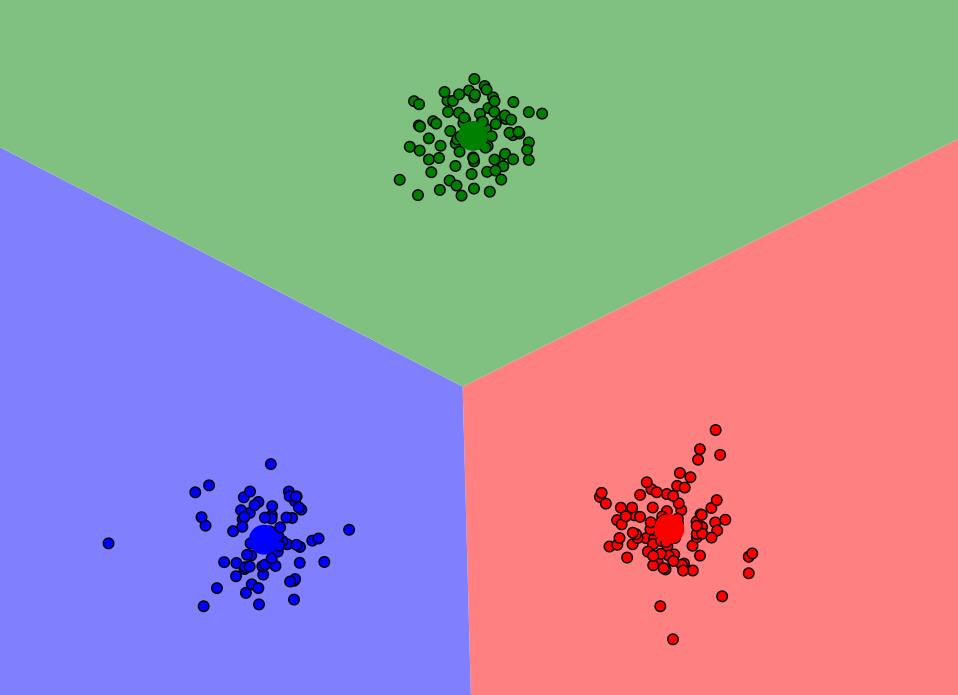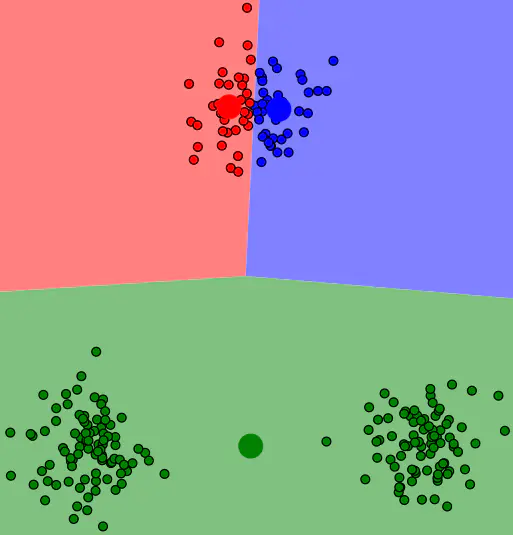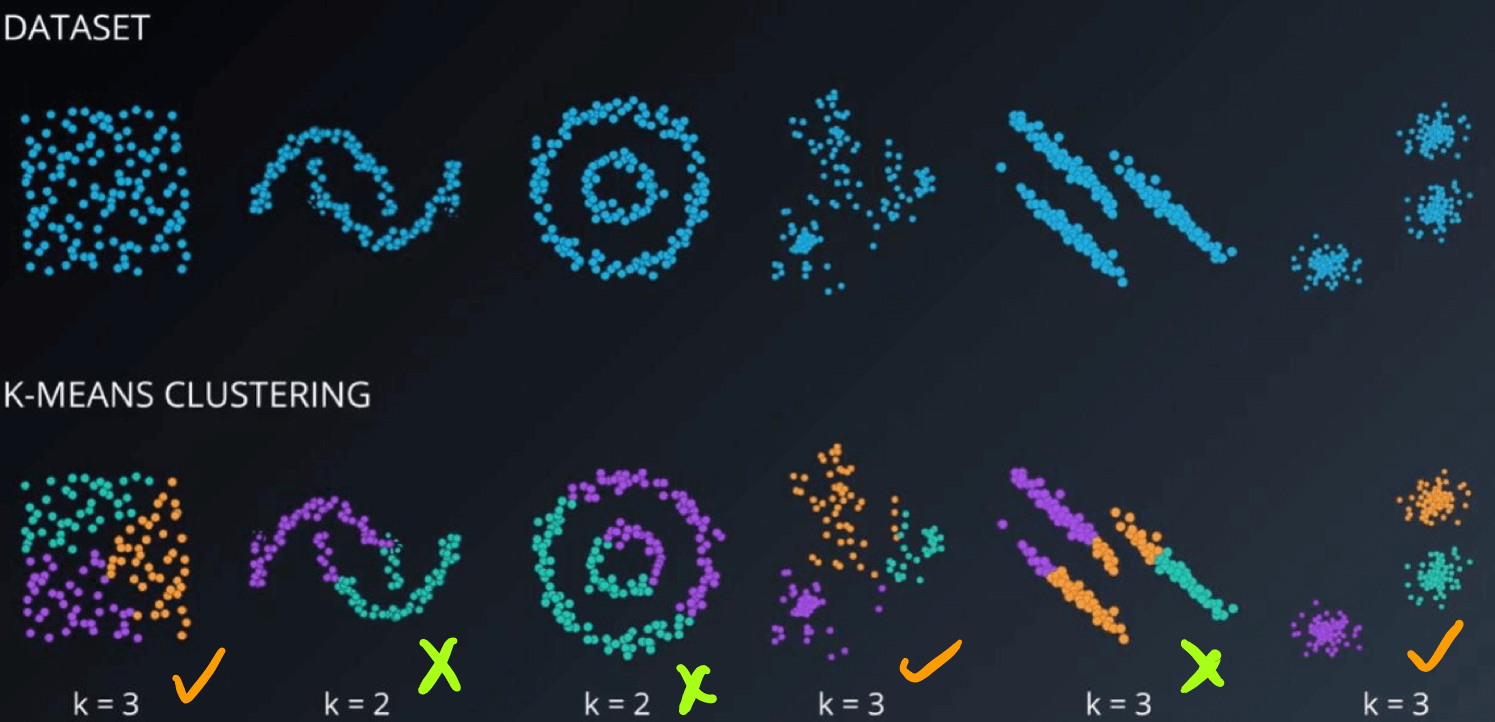# K-means

K-means是一种最为常用的硬聚类算法. 硬聚类指的是分出的样本必须只隶属于某一个类, 而不是给出隶属某几个类的概率.## 流程

$$E=\sum_{i=1}^{k} \sum_{\boldsymbol{x} \in C_{i}}\mid\mid\boldsymbol{x}-\boldsymbol{\mu}_{i}\mid\mid_{2}^{2}$$

$$\mu_i=\frac{1}{\mid C_i\mid}\sum_{\boldsymbol x \in C_i}\boldsymbol x$$

1. 从样本集中随机初始化, 随机选择$k$个样本集作为各类(簇)的中心点, $\{\mu_1,\mu_2,\dots, \mu_k\}$.
2. 计算所有样本点与各个簇中心之间的距离, 然后把每个样本点划入离该点最近的簇中.
3. 根据簇中已有的样本点, 重新计算簇中心$\mu_k$.
4. 重复2 - 3, 直到所有的点无法再更新到其他分类或达到最大迭代次数, 算法结束.

## 缺陷## 改进 K-means++

K-means++ 改进了原来的初始化中心点选取方法, 初始聚类中心相互之间应该分得越开越好.

1. 在数据点随机选取一个样本点为第一个簇中心$C_1$.
2. 计算剩余样本与所有簇中心的最短距离$D(x_i)=\min [dist(x_i, C_1), dist(x_i, C_2),\dots, dist(x_i, C_k)]$, 使得这个样本点被选为下一个簇中心的概率$P(x)=\frac{D(x)^{2}}{\sum_{x \in X} D(x)^{2}}$. 按照这个概率随机选取一个中心.
3. 重复步骤2直到选出$k$个簇中心.

## 适用性K-means只考虑簇中心(质心), 而不考虑簇的边缘和其他簇的关系, 所以不善于对狭长数据或质心位置与数据实际位置不着边的数据的聚类.

上一篇机器学习之随机森林

2020-08-15十大排序

2020-08-13
目录